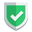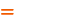我们相信：世界是美好的，你是我也是。平行空间的世界里面，不同版本的生活也在继续...

## 结巴提取标签的原理

``````import jieba.posseg
sentence = "苏南大叔最近心情很好，所以满世界瞎溜达。"
words = jieba.posseg.lcut(sentence)
print(words)``````

``[pair('苏南大叔', 'nr'), pair('最近', 'f'), pair('心情', 'n'), pair('很', 'd'), pair('好', 'a'), pair('，', 'x'), pair('所以', 'c'), pair('满', 'a'), pair('世界', 'n'), pair('瞎', 'v'), pair('溜达', 'v'), pair('。', 'x')]``

## 结巴分词提取标签函数

``````keywords=jieba.analyse.extract_tags(sentence)
print(keywords)``````

``['苏南大叔', '溜达', '心情', '最近', '世界', '所以']``

## 选择前几个标签

``````keywords=jieba.analyse.extract_tags(sentence, topK=3)
print(keywords)``````

``['苏南大叔', '溜达', '心情']``

## 显示标签权重

``````keywords=jieba.analyse.extract_tags(sentence, withWeight=True)
print(keywords)``````

``[('苏南大叔', 1.9924612504833332), ('溜达', 1.7391569582), ('心情', 1.0581609061033335), ('最近', 0.9612306317916667), ('世界', 0.727921567595), ('所以', 0.7053377915566666)]``

## 对词性进行筛选

``````keywords=jieba.analyse.extract_tags(sentence, allowPOS=("n","nr"))
print(keywords)``````

``['苏南大叔', '心情', '世界']``

## 最全参数的例子

``````keywords=jieba.analyse.extract_tags(sentence, topK=5, withWeight=True, allowPOS=())
print(keywords)``````

``[('苏南大叔', 1.9924612504833332), ('溜达', 1.7391569582), ('心情', 1.0581609061033335), ('最近', 0.9612306317916667), ('世界', 0.727921567595)]``

## 对带权重结果进行遍历

``````for w in keywords:
print(w,w)``````

``````list = [ w+str(w) for w in keywords ]
print(list)``````

## 最可能的seo使用方式

``````keywords=jieba.analyse.extract_tags(sentence, topK=5, allowPOS=("n","nr"))
print(keywords)``````

``['苏南大叔', '心情', '世界']``

``<meta name="keywords" content="">``

## 总结如果本文对您有帮助，或者节约了您的时间，欢迎打赏瓶饮料，建立下友谊关系。本博客不欢迎：各种镜像采集行为。请尊重原创文章内容，转载请保留作者链接。【福利】 腾讯云最新爆款活动！1核2G云服务器首年50元！【源码】本文代码片段及相关软件，请点此获取更多信息【绝密】秘籍文章入口，仅传授于有缘之人python    jieba